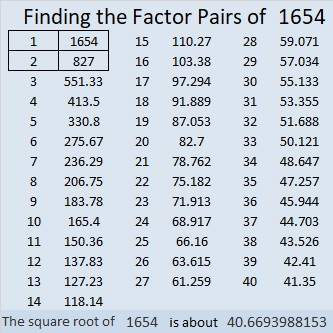# 1654 and Level 4

Contents

### Today’s Puzzle:

Use logic to write all the numbers 1 to 10 in both the first column and the top row of the puzzle so that those numbers are the factors of the given clues.### Factors of 1654:

• 1654 is a composite number.
• Prime factorization: 1654 = 2 × 827.
• 1654 has no exponents greater than 1 in its prime factorization, so √1654 cannot be simplified.
• The exponents in the prime factorization are 1 and 1. Adding one to each exponent and multiplying we get (1 + 1)(1 + 1) = 2 × 2 = 4. Therefore 1654 has exactly 4 factors.
• The factors of 1654 are outlined with their factor pair partners in the graphic below.### More About the Number 1654:

1654 is a leg in one Pythagorean triple:
1654-683928-683930, calculated from 2(827)(1), 827² – 1², 827² + 1².

This site uses Akismet to reduce spam. Learn how your comment data is processed.